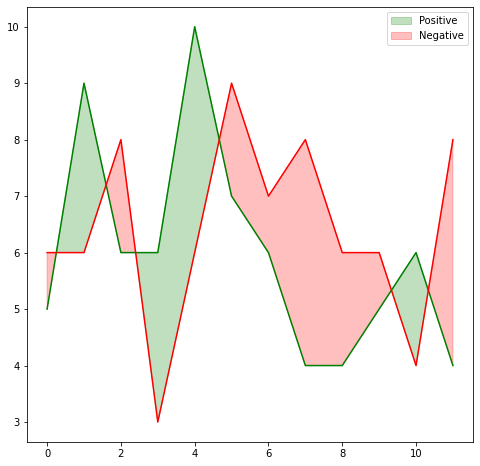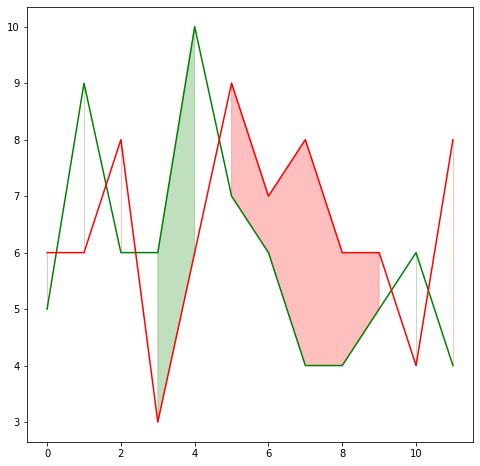# Area fill between two lines in Matplotlib

Once you know how to plot several lines with `Matplotlib` it's quite straightforward to add an area fill between them. This posts shows how to add an area fill between two lines with the color depending on which line has a larger value.

``````import matplotlib.pyplot as plt
import numpy as np``````

Let's get started by creating some fake data.

``````time = np.arange(12)
income = np.array([5, 9, 6, 6, 10, 7, 6, 4, 4, 5, 6, 4])
expenses = np.array([6, 6, 8, 3, 6, 9, 7, 8, 6, 6, 4, 8])``````

And now let's make the plot:

``````# Initialize figure and axis
fig, ax = plt.subplots(figsize=(8, 8))

# Plot lines
ax.plot(time, income, color="green")
ax.plot(time, expenses, color="red")

# Fill area when income > expenses with green
ax.fill_between(
time, income, expenses, where=(income > expenses),
interpolate=True, color="green", alpha=0.25,
label="Positive"
)

# Fill area when income <= expenses with red
ax.fill_between(
time, income, expenses, where=(income <= expenses),
interpolate=True, color="red", alpha=0.25,
label="Negative"
)

ax.legend();``````Note what's the result if `interpolate=False`:

``````# Initialize figure and axis
fig, ax = plt.subplots(figsize=(8, 8))

# Plot lines
ax.plot(time, income, color="green")
ax.plot(time, expenses, color="red")

# Fill area when income > expenses with green
ax.fill_between(
time, income, expenses, where=(income > expenses),
interpolate=False, color="green", alpha=0.25
)

# Fill area when income <= expenses with red
ax.fill_between(
time, income, expenses, where=(income <= expenses),
interpolate=False, color="red", alpha=0.25
);``````Setting `interpolate` to `True` calculates the intersection point between the two lines and extends the filled region up to this point, which results in the better looking filled area.

## Contact & Edit

👋 This document is a work by Yan Holtz. Any feedback is highly encouraged. You can fill an issue on Github, drop me a message onTwitter, or send an email pasting `yan.holtz.data` with `gmail.com`.